﻿ A Multilayer Perceptron Artificial Neural Networks Based a Preprocessing and Hybrid Optimization Task for Data Mining and Classification

A Multilayer Perceptron Artificial Neural Networks Based a Preprocessing and Hybrid Optimization Tas...

Kais Ncibi, Tarek Sadraoui, Mili Faycel, Amor Djenina

A Multilayer Perceptron Artificial Neural Networks Based a Preprocessing and Hybrid Optimization Task for Data Mining and Classification

Kais Ncibi1, Tarek Sadraoui2,, Mili Faycel2, Amor Djenina3

1University of Economics and Management of Sfax, MODILIS Lab

2University of Economics and Management of Mahdia, MODILIS Lab

3University of Tebessa Algerie

Abstract

Artificial neural networks (ANNs) optimization represent an attractive area that attract many researchers in different disciplines, this in the aim to improve the performance of this model. In literature, there is no fix theory that illustrates how to construct this non linear model. Thus, all proposed construction was based on empirical illustration. Multilayer perceptron (MLP) is one of the most used models in ANNs area. It was described as a good non linear approximator with a power ability to lean well non linear system, and most of research was limited to a 3 layers MLP, by describing that 3 layers are sufficient to have good approximation. In this context we are interested to this model construction for solving supervised classification tasks in data mining. This construction requires a preprocessing phase that seems to scribe be important for the final performance. This paper present a process of MLP construction based on two phases: a preparation phase and an optimization phase. The first one describes a process of data cleaning, discretization, normalization, expansion, reduction and features selection. The second phase aims to optimize the set of weights based on some combination of hybrid algorithms such back-propagation algorithm, a local search and different evolution. An empirical illustration will be done to in order to validate the proposed model. At the end, a comparison with others known classifiers will be done to justify the validity of the proposed model.

• Kais Ncibi, Tarek Sadraoui, Mili Faycel, Amor Djenina. A Multilayer Perceptron Artificial Neural Networks Based a Preprocessing and Hybrid Optimization Task for Data Mining and Classification. International Journal of Econometrics and Financial Management. Vol. 5, No. 1, 2017, pp 12-21. http://pubs.sciepub.com/ijefm/5/1/3
• Ncibi, Kais, et al. "A Multilayer Perceptron Artificial Neural Networks Based a Preprocessing and Hybrid Optimization Task for Data Mining and Classification." International Journal of Econometrics and Financial Management 5.1 (2017): 12-21.
• Ncibi, K. , Sadraoui, T. , Faycel, M. , & Djenina, A. (2017). A Multilayer Perceptron Artificial Neural Networks Based a Preprocessing and Hybrid Optimization Task for Data Mining and Classification. International Journal of Econometrics and Financial Management, 5(1), 12-21.
• Ncibi, Kais, Tarek Sadraoui, Mili Faycel, and Amor Djenina. "A Multilayer Perceptron Artificial Neural Networks Based a Preprocessing and Hybrid Optimization Task for Data Mining and Classification." International Journal of Econometrics and Financial Management 5, no. 1 (2017): 12-21.

 Import into BibTeX Import into EndNote Import into RefMan Import into RefWorks

12345
Prev Next

1. Introduction

Classification task is a very important topic in data mining. A lot of research [1, 2, 3] has focused on the field over the last two decades. Data mining is a knowledge discovery process from large databases. The extracted knowledge will be used by a human user for supporting a decision that is the ultimate goal of data mining. Therefore, classification decision is our aim in this study. A various classification models have been used in this regard. M. James  has employed a linear/quadratic discriminates techniques for solving classification problems. Another procedure has been applied using decision trees [5, 6]. In the same context, Duda et al.  have proposed a discriminate analysis based on the Bayesian decision theory.

Nevertheless, these traditional statistical models are built mainly on various linear assumptions that will be necessary satisfied. Otherwise, we cannot apply these techniques for classification tasks. To overcome the disadvantage, artificial intelligent tools have been emerged to solve data mining classification problems. For this purpose, genetic algorithms models were used . In a recent research, Zhang [9, 10] have introduced the neural networks technique as a powerful classification tool. In these studies, he showed that neural network is a promising alternative tool compared to various conventional classification techniques.

In this present research, we propose a MLP model based on a pre-processing and optimization phase. This model will be compared to other classifiers using different benchmark and real data bases.

At first, we will describe a same important concept that was important for the construction of our model. Next, we describe the proposed model. At the end, empirical validation will be presented

2. Concepts and Definition

2.1. Multilayer Perceptron

Multilayer Perceptron (MLP) is one of the most used ANNs, where 80% of ANNs researches focused on. It consists of a series of fully interconnected layers of nodes where there are only connections between adjacent layers. General structure is showed in Figure 1.Download asVeiw figureFigures index
Figure 1. A Fully connected multilayer perceptron (MLP)

The first layer (the input layer) takes as inputs the various attribute values. The output of the nodes in the input layer, multiplied with the weights attached to the links, is passed to the nodes in the hidden layer.

A hidden node collects the incoming weighted output values of the previous layers. Besides that, it receives also the weighted value of a bias node.

The sum of the weighted input values is passed through a nonlinear activation function. The only requirements are that the output values of the function are bounded to an interval and that the nonlinear function can be differentiable. The output of a node in the hidden layer is fed into the nodes of the output layer. To each node in the output layer a class label is assigned.

2.2. MLP Construction as an Optimization Problem

A MLP construction requires finding the best combination of weights, the best number of hidden layers and hidden nodes, and the best combination of relevant features.

2.2.1. The Best Combination of Weights

MLP optimization problem consist to find the best combination of weights that near real outputs and estimated ones. This problem is considered as a continuous optimization problem. Referring to the Classification of the optimization methods according to the nature of the problem, presented by Dreo, Jet Al. in 2005 , continuous optimization is represent one of problems difficult to optimize.Download asVeiw figureFigures index
Figure 2. Optimization methods classification

2.2.2. The Best Number of Hidden Layers and Hidden Nodes

Other than finding weights, a MLP construction problem is o find the number of hidden layers and hidden nodes in each layer. In most literature, we find that MLP with one hidden layer can be considered as a universal approximated for each non-linear function with a strong predictive ability. So, in our study, MLP with one hidden layer will be considered.

So we ask the question” how to find the number of hidden nodes in this layer?” In order to simplify this task, many researches take a prior fixed number of hidden nodes. For example, they take this number equal to the number of features, or equal to the half of this number. But this prior fixation can give negative effect on the final performance.

Referring to , we propose to use a prior test in order to fix the number of hidden nodes. The idea if to begin from a small number of hidden nodes and growing until reaches a prior fixed maximum number of hidden nodes. But is same case, to be located in a high number of hidden nodes can result the over fitting problem. So, in this work, we propose to fix the number of hidden nodes and add an expansion function in order to maximize the representatively of non linear data.

2.2.3. Select The Best Combination of Relevant Features

An important issue in classification is how many features to use when training a MLP. Including “irrelevant” attributes will result a negative effect on the performance and a slowly execution. Moreover, measuring redundant attributes costs time and money. Many techniques are presented to feature selection task. A simple technique is to execute simple Statistical the paired-t-test, and remove non significant features. But this technique has some linearity discrimination and can remove some features that help to improve results. For this aim we include mutual information and function expansion in order to represent better initial features.

2.3. Features Selection Problem Based Prior Test

Statistical tests can be used in order to evaluate each input feature and decide if it will be used later or it will be deleted.

We suppose that we have two hypotheses.

H0: The feature is not relevant (have the same means in each class groups).

H1: Classifiers are different (means in each class groups is different).

To test the null hypothesis, the procedure is to compute the t-statistic and to compare it to the tabulated T. We accept H1 if and only if: the computed T > the tabled T. We can use the p-value for the evaluation. That means we accept H1 if and only if: P-value < standard level of significance.

In most cases, the standard level of significance is taken equal to 5%. We can use this level equal to 10% if we like to be tolerable with features, or 1% if we like to execute strong removing task.

In our study, this level will be fixed based on prior experimental test.

2.4. Receiver Operating Characteristic (ROC) Curve, Area under the Curve (AUC)

It is a measure of the accuracy of the model. It ranks the test sample in decreasing order: the one that is most likely to belong to the positive class appears at the top of the list. The closer to the diagonal line, the less accurate is the model. More the AUC is near 1 more the classifier have a good ability to discriminate well between the two classes.

2.5. Mutual Information

In information theory, mutual information measures the statistical dependence between two random variables and so can be used to evaluate the relative utility of each feature to classiﬁcation. It is defined as: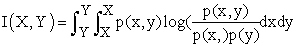Where p(x, y) is the joint probability density function of continual random variables X and Y, and p(x) and p(y) are the marginal probability density functions respectively. It is not difficult to ﬁnd that mutual information is related to entropy as: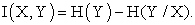Given the Shannon entropy (discrete) deﬁned as: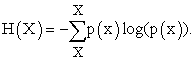The use of mutual information for feature selection can be intuitively justiﬁed. From the point of view of statistics, the mutual information measures more general dependency in the data, and therefore may lead to better capability to feature selection.

2.6. Features Selection Problem

Feature selection is one the important tasks in data mining area. As presented in Figure 8 Kohavi and John G.  classify features into 3 fundamental classes: class of strong features, class of weak features and class of irrelevant features. Last ones must be removed from the model in order to improve the performance of the model and makes the optimization process faster.

Strong features must be used in the classification process, because these features separate well between classes. A part of weak features can be conserved if they improve the performance of our model.Download asVeiw figureFigures index
Figure 3. Relevance of a feature

Referred to R. Kohavi and John G. , feature selection algorithms can be grouped into two categories: filter approach and wrapper approach:

• The first one uses a classification algorithm that automatically performs variable selection as part of the definition of the basic models such decision tree classifier.

• The second approach uses classifier as a black-box and has an external loop named wrapper that systematically adds variables to the current subset, and each subset being evaluated on the basis of how well the classification model performs.

In this work, we propose a prior constructive process based on different features selections tools. Detailed description of this model will be done next.

3. Model Description

We propose a constructive ANN model for classification task this model is composed with 2 phases

• A preprocessing phase:

• An optimization phase:

A detailed description of these phases will be illustrated next:

3.1. A Preprocessing Phase

The preprocessing phase is described in Figure 1. This phase prepare initial data for the optimization one, It is an important task that can influence final result of the proposed model. These steps are described as follows:

3.1.1. Data Cleaning

It is one of important tasks in data warehouse task. In our model we are limited to outlier’s adjustment. An observation is described as an outlier if it is out of some boundary. For example, we can use the interval inter-quartile IIQ, and we can describe an outlier as a point out of 1.5*IIQ.

• If this observation is identified, we propose to use regression as follows

• Select the 2 best most correlated features to the correspondent one

• Construct a linear model using the features

• Predict the new value of the outlier and replace the old one

The proposed outliers control can be used for missing data.

3.1.2. Feature Discretization

Discretization can be a good task for data representation. Data can be more comprehensive, ANN became faster ad and rules extraction tasks can be executed. Else some evaluation criteria for input features can be computed such mutual information criteria.

3.1.3. Data Expansion

While we aim to use ANN, as a non linear approximator, we propose to use expansion function in order to represent better no linearity of the data. Many expansion function was found such the trigonometric, the polynomial, the Legendre polynomial, the power series, and the exponential and the logarithmic transformation. We propose to use many expansions functions in the same time and select the best one based on the principle of mutual information.

3.1.4. Data Reduction

Clustering can be a good alternative for the proposed model. It can reduce the number of features, the required time for convergence and it can improve performance and required time. We propose to use the principal component analysis techniques (PCA) and the self organizing mapping (SOM). These two techniques are very used for clustering task. After prior experiences, we select the PCA as a principal clustering technique, and it is proved to be more compatible for our model.

3.1.5. Feature Selection

Feature selection problem is to select a minimum set of features that perform well the performance of the studied model. In literature, many techniques can be found such, heuristic methods as Step-wise forward selection, Step-wise backward elimination, and decision tree induction. We propose preprocessing approach, based on t-tests, correlation coefficient, and mutual information.

3.1.6. Data Normalization

We use the mix-max normalization function, we choose to use logsig transfer function and the normalization between [0, 1]. This one is the best recommended for ANN models.

3.1.7. Data Division and Cross-validation

K-fold cross-validation re-sampling technique is one of the most know technique that can be used in classification in order to avoid over fitting. In this study, 10-fold cross-validation re-sampling technique is used. 80% of data is used for training, 10% for validation and 10% for the test. Experience will be repeated 10 times, and at each time, we change the test fold and the validation fold.Download asVeiw figureFigures index
Figure 4. The pre-processing phase of an ANN construction
3.2. An Optimization Phase

The optimization phase of an ANN construction has the aim to find the best combination of weight that improves better performance function. This problem is classed as a continued non linear optimization problem, and it is hard to be optimized. Many algorithms are found in literature. The most used one is the back-propagation algorithm. The last one performs good results, but it can be confronted in a problem of local minimum. For this aims, we add a local search algorithm and a differential evolution algorithms, in the hope to avoid this problem and increase the chance of faster convergence.

A detailed description of the optimization phase is presented in Figure 2. This phase is illustrated as follows:

3.2.1. Initial Weight and Improving Initial Weights

The process begins by generating randomly initial weights. We generate 10000 times weights and we select the best one of these generation based on the criteria of minimum MSE.

3.2.2. Apply the Back-propagation Algorithm

This algorithm is used at first as a basic optimization algorithm of the model.

3.2.3. Apply the Local Search

After applying the back-propagation algorithms, we add to each weight a random walk and we observe the MSE effect. Weight will be updated if adding this random walk improves the MSE function.

3.2.4. Apply the Differential Evolution

Differential evolution is an evolutionary algorithm that can have a good ability to improve our weights. If we suppose that we have 3 states of weights:

W1: initial weights

W2: solution weights after the back propagation process.

Wbest: is the set of weights after applying local search

Many schemes can be found in literature. While we have only 3 states of weights, we choose the following scheme DE/best/1. This schema updates weights as follows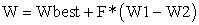Where F define the constriction factor generally taken equal to 0.5.Download asVeiw figureFigures index
Figure 5. The optimization phase of an ANN construction

3.2.5. Model evaluation

3 basic functions are choose for evaluation such

The mean square error function: noted MSE.

The misclassification function: noted MCE.

The area under the ROC curve: noted AUC.

The criteria that will use for comparison is the MSE. The MCE and the AUC are just displayed to observe their dynamic evolution over the dynamic process.

Others evaluation criteria can be used such:

The required time: Time can be a good alternative for comparison. More we are faster, more we takes quick decision.

Mean of mutual information: each model can have different form if input features, the mean of mutual information of these features can be a good alternative to observe model before and after features transformation. The last one can be resulted by clustering, expansion, features removing etc.

The number of improvement: When dealing with hybrid algorithms, we need to know which algorithm improve better than. In this case we implement an instruction the count the number of improvement of each algorithm. 3 number of improvement are defined such:

• Number of improvement of back-propagation

• Number of improvement of local search

• Number of improvement of differential evolution.

3.2.6. Stopping Criteria

The process will run in a cycle while there is MSE improvement. Stopping criteria is an instruction that fix where our process must be stopped. 3 stopping criteria are fixed:

We reach a maximum number of epochs

We fix this number equal to 20 for prior test, and 100 for the final model optimization.

Reaching a MSE less than a prior fixed level

We fix MSE level equal to 0,001.

Having a number of successive execution without MSE improvement

We fix this number level equal to 5 executions.

4. Experimental Results

8 real-world databases were selected there to be used in simulation works. 6 are chosen from the UCI repository machine learning, which is commonly used to benchmark learning algorithms . The “Tunisian Breast Cancer” Database was collected from real Tunisian hospital. The “Islamic vs conventional bank” database were extracted from the “banqscop” database source .

4.1. Databases Description

A brief description of used databases for experimental setup is presented in Table 1. Num. is the numeric features, Bin. is the binary ones, and Nom. is the nominal inputs that mean discrete with three or more distinct labels.

Table 1. Summary of the dataset used in simulation studies

4.2. Experiences Description

The experience consists to run 10 times the proposed process over all the 8 presented databases. For each database, we compute the mean of each performance evaluation criteria. Next, we compute the mean of the 8 means of each database.

Based on the training set, the validation set and the test set, 20 first epochs will be observed. Results will be displayed based on

• MSE, MCE, AUC,

• The number of improvement of BP, LS and DE,

• The mean of mutual information

• The required time for execution.

At first we display results of the optimization phase without any preprocessing task. Next we display results with the total proposed process.

Tables and figures will displays MSE, MCE and AUC using this transformation.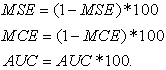These transformations were done in order to display the 3 criteria in the same figure.

4.3. Convergence of the Proposed Model

Based on the MSE function, and in order to observe the convergence of our model over 50 run epochs, we used the ‘breast cancer database”. The last one was taken from the UCI repository machine learning . Results are presented in the next figure.

From this figure, we can approve the strong ability of our model to converge quickly to a better MSE results. So, the convergence process is confirmed.Download asVeiw figureFigures index
Figure 6. MSE, MCE and AUC Convergence of the database process using the breast cancer database
4.4. Comparing Initial and Final Constructed Model

Table 2 shows results of the optimization phase and the total proposed process. By comparing the two results, we can observe the total improvement of this performance functions. This improvement is presented in Figure 7.Download asVeiw figureFigures index
Figure 7. MSE MCE AND AUC of initial and final model, after 20 epochs

By comparing the number of improvement, as shown in Figure 8, the execution of a good pre-processing phase improve the number of improvement of the back-propagation and the local search algorithm.Download asVeiw figureFigures index
Figure 8. Number of imporvement of BP, LS and DE before and after preprocessing execusion

Now, wa compare the mean of mutual information, and we observe that this criteria pass from 0.27 to 0.40. So the preprocessing phase contributes to improve mutual information. This result is shown in Figure 8.

Figure 10 shows the required time to execute the total process. We observe an improvement for the required time. The last one pass from 1611 to 1561.Download asVeiw figureFigures index
Figure 9. Mutual information evolution before and after the preprocessing execusionDownload asVeiw figureFigures index
Figure 10. Required time for execusion with and without a pre-processing results
4.5. Comparing Results to Other Classifiers

A basic comparison between the proposed model and 5 others classifiers is performed using 9 databases. These classifiers are:

• The K-nearest Neighbor (KNN),

• The support vector machine (SVM),

• The decision tree based C.45,

• The multilayer perceptron (MLP) based back-propagation algorithms

Results are presented in Table 3. From this table, and compared to others classifiers, we find that our model performance is better for all tested databases. We say that this model is a good classifier and it gives better and significant results.

Table 3. The proposed model vs other classifiers

Figure 11 shows that the correct rate of classification of the proposed model is 88, 88%. And this rate is the best one.Download asVeiw figureFigures index
Figure 11. The rate of correct classification of the proposed model vs other classifiers

Figure 12 shows that the AUC of classification of the proposed model is 87, 63%. Which is the best too?Download asVeiw figureFigures index
Figure 12. The AUC of the proposed model vs other classifiers

Figure 13 shows that the proposed process is slower, if it is compared to others classifiers.Download asVeiw figureFigures index
Figure 13. The required of the proposed model vs other classifiers

5. Conclusion

A constructive ANN was proposed was based on two fundamental phases, a pre-processing phase and an optimization phase. This classifier shows his ability to converge well and gives good results.

Compared to others classifiers, our model give the best results of correct rate of classification and AUC. But it require more times and it is the most slower one

In future work, we can include a wrapper approach able to delete automatically irrelevant features. We can also find a process of rule extraction in order to perform the comprehensibility of our classifier.

Others instruction can be included in the proposed process in order to perform better speed of execution.

References

  R. Agrawal, T. Imielinski and A. Swami. “Database mining: A performance perspective”. IEEE Trans. Knowledge Data Eng., 5, pp. 914-925 (1993).In article View Article  U. M. Fayyad, Piatetsky-Shapiro, G., and Smyth, P.. “From data mining to knowledge discovery: An overview”. In U. M. Fayyad, G. Piatetsky-Shapiro, & P. Smyth (Eds.), Advances in knowledge discovery and data mining ,pp. 1–34,. Menlo Park, CA: AAAI Press, 1996.In article  H.P. Kriegel, et al.. “Future trends in data mining”. Data Mining and Knowledge Discovery, 15(1), 87–97. Netherlands: Springer (2007).In article PubMed  M., James. “Classification Algorithms”. Wiley, 1985.In article  L. Breiman,, J.H. Friedman, R.A. Olshen and C.J. Stone. “Classification and Regression Learning”. Morgan Kaufman, 1984.In article  H.P. Kriegel, et al.. “Future trends in data mining”. Data Mining and Knowledge Discovery, 15(1), 87-97. Netherlands: Springer (2007).In article PubMed  R.O. Duda, Hart PE and Stork D.G.. “Pattern classification”. Wiley, New York, 2001.In article  D.E. Goldberg. “Genetic algorithms in search, optimization and machine learning”. Morgan Kaufmann, 1989.In article  G. P., Zhang. ”Neural networks for classification”, A survey. IEEE Transactions on Systems, Man, Cybernetics-Part C: Application and Reviews, 30(4), pp. 451-461, 2000.In article  G. P Zhang. “Avoiding pitfalls in neural network research”. IEEE Transactions on Systems, Man and Cybernetics-Part C: Applications and Reviews, 37(1), pp. 3-16, 2007.In article View Article  Feraud, R. and CLEROT, F.. A Methodology to Explain Neural NetworkClassification. Neural Networks, 15, 2002, 237-246.In article View Article  Sarle, W.S.: How to measure the importance of inputs?. Technical report, SAS Institute Ins, Cary, NC, USA. ftp://ftp.sas.com/pub/neural/FAQ.html,1998.In article  Dreo, J., Petrowski, A., Siarry, P., Tailard, E.. Metaheuristic for Hard Optimization: Methods and Case Studies. Springer, Heidelberg, 2005.In article  Craven M. W. and Shavlik J.W.. Extracting Tree structured representation of trained neural network, Neural information processing systems, (8), 24-30 (1996).In article  Kohavi R., and G. John (1997). Wrappers for feature subset selection, Artificial Intelligence, vol. 97, no. 1-2, pp. 273-324.In article View Article  L., Fogel, J. Owens and J. Walsh, “Artificial Intelligence through Simulated Evolution”. John Wiley, Chichester, 1966.In article  D.E. Goldberg. “Genetic algorithms in search, optimization and machine learning”. Morgan Kaufmann, 1989.In article  J., Holland. “Adaptation in natural and artificial systems”. Univ. of Michigan Press, Ann Arbor, 1975.In article  J. Koza,. “Genetic programming on the programming of computers by means of natural selection”, Cambridge MA: MIT Press, 1992.In article PubMed  M. Dorigo,, Stutzle, T., ”Ant Colony Optimization”. MIT Press, Cambridge., 2004.In article  J., Kennedy Eberhart R., “Particle Swarm Optimization”, In Proceedings of IEEE International Conference on Neural Networks, pp. 1942-1948, 1995.In article View Article  R. Storn Kenneth P. “Differential evolution A simple and efficient adaptive scheme for global optimization over continuous spaces”. Technical Report TR, pp. 95-012, International Computer Science Institute, Berkeley, CA, 1995.In article  F. Hui-Y. and J. Lampinen,“A trigonometric mutation approach to differential evolution”. In K. C. Giannakoglou, D. T. Tsahalis, J. Periaux, K. D. Papailiou, & T. Fogarty, editors, Evolutionary Methods for Design Optimization and Control with Applications to Industrial Problems, pp. 65–70, Athens, Greece. International Center for Numerical Methods in Engineering (Cmine), 2001.In article  Y.-H. Pao, S.M. Phillips and D.J. Sobajic, “Neural-net computing and intelligent control systems”. Int. J. Contr., 56, pp. 263-289, 1992.In article View Article  R. Majhi, G. Panda and G. Sahoo, “Development and performance evaluation of FLANN based model for forecasting of stock markets”, Expert Systems with Applications 36, pp. 6800-6808, 2009.In article View Article  C.L. Blake,. and Merz, C.J., ‘UCI Repository of machine learning databases’, em Irvine, CA: University of California, department of information and Computer Science. Available on-line at: http//www.ics.uci.edu/~mlearn/ML.Repository.html., 1998.In article